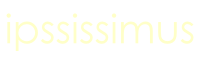Sacred GeometrySaturday 9th December -

Home# Pentacle

AB is the starting length. Set compasses to this length and draw a pair of circles centres A and B. Join the intersection points C and D with a line. Draw a circle centre C. Label the point where the circle intersects CD as O.Label the points where this circle intersects the circles with centre A and the circle with centre B as E and F. Join E to O and continue the line to intersect the circle with centre B at G. Now join F to O and continue the line to intersect the circle with centre A at H. Draw circle with centres G and H - label the point of intersection as I. Now join ABGIH to form a pentacle.# Septacle/Heptagon

Find A, a random point on the circle. Find M, the midpoint of OA.Draw a perpendicular line through M. It intersects circle O at B. Draw a circle at B so that it crosses M. Circle B intersects circle O at two points of the heptagon. You can use them to find the rest and join the points as in diagram.